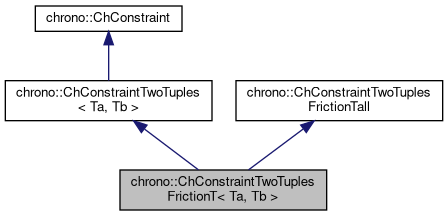chrono::ChConstraintTwoTuplesFrictionT< Ta, Tb > Class Template Reference

## Description

### template<class Ta, class Tb> class chrono::ChConstraintTwoTuplesFrictionT< Ta, Tb >

Base class for friction constraints between two objects, each represented by a tuple of ChVariables objects.

This constraint cannot be used alone. It must be used together with a ChConstraintTwoTuplesContactN

#include <ChConstraintTwoTuplesFrictionT.h>

Inheritance diagram for chrono::ChConstraintTwoTuplesFrictionT< Ta, Tb >:[legend]
Collaboration diagram for chrono::ChConstraintTwoTuplesFrictionT< Ta, Tb >:[legend]

## Public Member Functions

ChConstraintTwoTuplesFrictionT ()
Default constructor.

ChConstraintTwoTuplesFrictionT (const ChConstraintTwoTuplesFrictionT &other)
Copy constructor.

virtual ChConstraintTwoTuplesFrictionTClone () const override
"Virtual" copy constructor (covariant return type).

ChConstraintTwoTuplesFrictionToperator= (const ChConstraintTwoTuplesFrictionT &other)
Assignment operator: copy from other object.

virtual bool IsLinear () const override
Indicate whether or not this constraint is linear.

virtual double Violation (double mc_i) override
The constraint is satisfied?Public Member Functions inherited from chrono::ChConstraintTwoTuples< Ta, Tb >
ChConstraintTwoTuples ()
Default constructor.

ChConstraintTwoTuples (const ChConstraintTwoTuples &other)
Copy constructor.

ChConstraintTwoTuplesoperator= (const ChConstraintTwoTuples &other)
Assignment operator: copy from other object.

type_constraint_tuple_a & Get_tuple_a ()
Access tuple a.

type_constraint_tuple_b & Get_tuple_b ()
Access tuple b.

virtual void Update_auxiliary () override
This function must update jacobians and auxiliary data such as the 'g_i' product. More...

virtual double Compute_Cq_q () override
This function must computes the product between the row-jacobian of this constraint '[Cq_i]' and the vector of variables, 'v'. More...

virtual void Increment_q (const double deltal) override
This function must increment the vector of variables 'v' with the quantity [invM]*[Cq_i]'deltal,that is v+=[invM][Cq_i]'*deltal or better: v+=[Eq_i]*deltal This is used for some iterative solvers.

virtual void MultiplyAndAdd (double &result, const ChVectorDynamic< double > &vect) const override
Computes the product of the corresponding block in the system matrix by 'vect', and add to 'result'. More...

virtual void MultiplyTandAdd (ChVectorDynamic< double > &result, double l) override
Computes the product of the corresponding transposed blocks in the system matrix (ie. More...

virtual void Build_Cq (ChSparseMatrix &storage, int insrow) override
Puts the two jacobian parts into the 'insrow' row of a sparse matrix, where both portions of the jacobian are shifted in order to match the offset of the corresponding ChVariable.The same is done on the 'insrow' column, so that the sparse matrix is kept symmetric.

virtual void Build_CqT (ChSparseMatrix &storage, int inscol) override
Same as Build_Cq, but puts the transposed jacobian row as a column.Public Member Functions inherited from chrono::ChConstraint
ChConstraint ()
Default constructor.

ChConstraint (const ChConstraint &other)
Copy constructor.

ChConstraintoperator= (const ChConstraint &other)
Assignment operator: copy from other object.

bool operator== (const ChConstraint &other) const
Comparison (compares only flags, not the jacobians etc.)

bool IsValid () const
Tells if the constraint data is currently valid.

void SetValid (bool mon)
Use this function to set the valid state (child class Children classes must use this function depending on the result of their implementations of RestoreReference();.

bool IsDisabled () const
Tells if the constraint is currently turned on or off by the user.

void SetDisabled (bool mon)
User can use this to enable/disable the constraint as desired.

bool IsRedundant () const
Tells if the constraint is redundant or singular.

void SetRedundant (bool mon)
Solvers may use the following to mark a constraint as redundant.

bool IsBroken () const
Tells if the constraint is broken, for excess of pulling/pushing.

void SetBroken (bool mon)
3rd party software can set the 'broken' status via this method (by default, constraints never break);

virtual bool IsUnilateral () const
Tells if the constraint is unilateral (typical complementarity constraint).

eChConstraintMode GetMode () const
Gets the mode of the constraint: free / lock / complementary A typical constraint has 'lock = true' by default.

void SetMode (eChConstraintMode mmode)
Sets the mode of the constraint: free / lock / complementary.

bool IsActive () const
Tells if the constraint is currently active, in general, that is tells if it must be included into the system solver or not. More...

void SetActive (bool isactive)
Set the status of the constraint to active.

virtual double Compute_c_i ()
Compute the residual of the constraint using the LINEAR expression. More...

double Get_c_i () const
Return the residual 'c_i' of this constraint. // CURRENTLY NOT USED.

void Set_b_i (const double mb)
Sets the known term b_i in [Cq_i]*q + b_i = 0, where: c_i = [Cq_i]*q + b_i = 0.

double Get_b_i () const
Return the known term b_i in [Cq_i]*q + b_i = 0, where: c_i= [Cq_i]*q + b_i = 0.

void Set_cfm_i (const double mcfm)
Sets the constraint force mixing term (default=0). More...

double Get_cfm_i () const
Returns the constraint force mixing term.

void Set_l_i (double ml_i)
Sets the 'l_i' value (constraint reaction, see 'l' vector)

double Get_l_i () const
Return the 'l_i' value (constraint reaction, see 'l' vector)

double Get_g_i () const
Return the 'g_i' product , that is [Cq_i]*[invM_i]*[Cq_i]' (+cfm)

void Set_g_i (double m_g_i)
Usually you should not use the Set_g_i function, because g_i should be automatically computed during the Update_auxiliary() .

virtual void Project ()
For iterative solvers: project the value of a possible 'l_i' value of constraint reaction onto admissible orthant/set. More...

void SetOffset (int moff)
Set offset in global q vector (set automatically by ChSystemDescriptor)

int GetOffset () const
Get offset in global q vector.

virtual void ArchiveOUT (ChArchiveOut &marchive)
Method to allow serialization of transient data to archives.

virtual void ArchiveIN (ChArchiveIn &marchive)
Method to allow de-serialization of transient data from archives.Protected Attributes inherited from chrono::ChConstraintTwoTuples< Ta, Tb >
type_constraint_tuple_a tuple_a

type_constraint_tuple_b tuple_bProtected Attributes inherited from chrono::ChConstraint
double c_i
The 'c_i' residual of the constraint (if satisfied, c must be 0)

double l_i
The 'l_i' lagrangian multiplier (reaction)

double b_i
The 'b_i' right term in [Cq_i]*q+b_i=0 , note: c_i= [Cq_i]*q + b_i.

double cfm_i
The constraint force mixing, if needed (usually is zero) to add some numerical 'compliance' in the constraint, that is the equation becomes: c_i= [Cq_i]*q + b_i + cfm*l_i =0; Example, it could be cfm = [k * h^2](^-1) where k is stiffness.

eChConstraintMode mode
mode of the constraint: free / lock / complementar

double g_i
'g_i' product [Cq_i]*[invM_i]*[Cq_i]' (+cfm)

int offset
offset in global "l" state vector (needed by some solvers)

The documentation for this class was generated from the following file:
• /builds/uwsbel/chrono/src/chrono/solver/ChConstraintTwoTuplesFrictionT.h